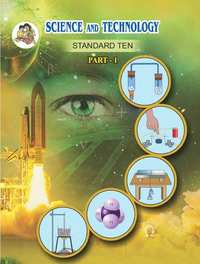SSC (English Medium) Class 10th Board ExamMaharashtra State Board
Share

# Balbharati solutions for Class 10th Board Exam Science and Technology - 1 chapter 6 - Refraction of light

## Balbharati Class 10 Science and Technology part 1

#### Balbharati Balbharati Class 10 Science and Technology part 1## Chapter 6: Refraction of light

#### Chapter 6: Refraction of light solutions [Page 79]

Q 1.1 | Page 79

Fill in the blanks and Explain the completed sentences.
a . Refractive index depends on the ............. of light.

Q 1.2 | Page 79

Fill in the blanks and Explain the completed sentences.

b. The change in ................ of light rays while going from one medium to another is called refraction.

Q 2.1 | Page 79

Prove the statement .
a. If the angle of incidence and angle of emergence of a light ray falling on a glass slab are i and e respectively, prove that, i = e.

Q 2.2 | Page 79

Prove the statement.

A raibow is the combined effect of the refraction, dispersion, and total internal reflection of light.

Q 3.1 | Page 79

Mark the correct answer in the questions.
A. What is the reason for the twinkling of stars?

• i . Explosions occurring in stars from time to time

• ii. Absorption of light in the earth’s atmosphere

• iii. Motion of stars

• iv. Changing refractive index of the atmospheric gases

Q 3.2 | Page 79

Mark the correct answer in the questions.

We can see the Sun even when it is little below the horizon because of

• i. Reflection of light

• ii. Refraction of light

• iii. Dispersion of light

• iv. Absorption of light

Q 3.3 | Page 79

Mark the correct answer in the  questions.

If the refractive index of glass with respect to air is 3/2, what is the refractive index of air with respect to glass?

• a. $\frac{1}{2}$

•  b. 3

• c. $\frac{1}{3}$

•   d. $\frac{2}{3}$

Q 4.1 | Page 79

Solve the examples.
a. If the speed of light in a medium is 1.5 × 108 m/s, what is the absolute refractive index of the medium?

Q 4.2 | Page 79

Solve the examples.

If the absolute refractive indices of glass and water are 3/2 and 4/3 respectively, what is the refractive index of glass with respect to water?

## Chapter 6: Refraction of light

#### Balbharati Balbharati Class 10 Science and Technology part 1## Balbharati solutions for Class 10th Board Exam Science and Technology - 1 chapter 6 - Refraction of light

Balbharati solutions for Class 10th Board Exam Science and Technology - 1 chapter 6 (Refraction of light) include all questions with solution and detail explanation. This will clear students doubts about any question and improve application skills while preparing for board exams. The detailed, step-by-step solutions will help you understand the concepts better and clear your confusions, if any. Shaalaa.com has the Maharashtra State Board Balbharati Class 10 Science and Technology part 1 solutions in a manner that help students grasp basic concepts better and faster.

Further, we at Shaalaa.com provide such solutions so that students can prepare for written exams. Balbharati textbook solutions can be a core help for self-study and acts as a perfect self-help guidance for students.

Concepts covered in Class 10th Board Exam Science and Technology - 1 chapter 6 Refraction of light are Refraction of Light, Laws of Refraction, Refractive Index, Twinkling of Stars, Dispersion of Light, Partial and Total Internal Reflection.

Using Balbharati Class 10th Board Exam solutions Refraction of light exercise by students are an easy way to prepare for the exams, as they involve solutions arranged chapter-wise also page wise. The questions involved in Balbharati Solutions are important questions that can be asked in the final exam. Maximum students of Maharashtra State Board Class 10th Board Exam prefer Balbharati Textbook Solutions to score more in exam.

Get the free view of chapter 6 Refraction of light Class 10th Board Exam extra questions for Science and Technology - 1 and can use Shaalaa.com to keep it handy for your exam preparation

S